Institute of Solid State Physics, University of Latvia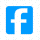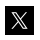ENG   LV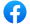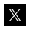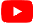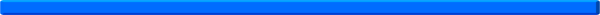Electron binding energies, in electron volts. (In PDF format) Physical constants. (In PDF format) Properties of the elements. (In PDF format) Periodic Table of Elements. (In PDF format) Crystal and ionic radii of the elements. (In PDF format) 1 meter = 103 millimeters = 106 micrometers = 109 nanometers = 1012 picometers = 1015 femtometers 1 THz = 33.36 cm-1; 1 meV = 8.066 cm-1; 1 Hartree = 27.2116 eV More units conversion.